# 18 – The Lie of the Land

 < ρℝεν | ℂσητεητs | ℕεχτ >“I was overwhelmed by the richness and beauty of the geometric ideas flowing from Lie’s work. He thought and wrote in grandiose terms, in a style that has now gone out of fashion, and that would be censored by our scientific journals!” – Robert Hermann in the preface to: Lie Groups : History, Frontiers and Applications

Over the course of the next three Chapters we will meet two new and related concepts, Lie Algebras and Lie Groups. Way back in Chapter 2 I wrote the following:

If you look at the Wikipedia entry for Special Unitary Groups you quickly end up disappearing down a rabbit-hole. The initial definition is as follows:

 “The special unitary group of degree n, denoted SU(n), is the Lie group of n×n unitary matrices with determinant 1”

A number of questions naturally arise:

1. What is a Group?
2. What does “unitary” mean?
3. What is a Lie Group?
4. What are matrices?
5. What is a determinant?

In the intervening Chapters we have answered all of the above questions save for “What is a Lie Group?”. Here we will begin to remedy this omission; although the question will not be directly answered until we reach the next Chapter and this initial answer will also be expanded upon in Chapter 20. It is a bit of a toss up whether to discuss Lie Groups and then move on to the related Lie Algebras, or vice versa . I have decided to look at Lie Algebras first and it is Lie Algebras that are the subject of both this Chapter and part of the next. Here the material we covered on Vector Spaces in Chapter 15 and Chapter 16 will be helpful because a Lie Algebra is a special type of Vector Space. Lie Algebras and Lie Groups are intimately connected and we will explore their relationship in more detail in Chapter 19 and Chapter 20.

Getting Crotchety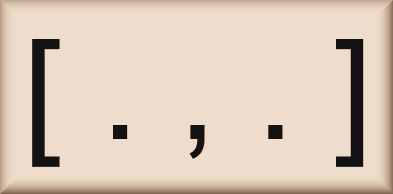In the previous Chapter we defined the ten properties that a structure must possess in order to be a Vector Space. Lie Algebras are Vector Spaces, but ones with some additional properties as well. In particular, if we have a Vector Space, V, then in order for it to become a Lie Algebra, it must have another binary operator acting on it (sometimes called either a multiplication or product) which is called the Lie Bracket. The Lie Bracket is denoted by square brackets, so for any two v, u ∈ V, the Lie Bracket is a mapping from V × V into V such that (v, u) ↦ [v, u].

If we further assume that our Vector Space, V, is over a Field F, then the Lie Bracket must satisfy a further three properties as follows:

1. If v ∈ V then:

[v, v] = 0

This property is known as Alternativity.

2. If v, u, w ∈ V and f, g ∈ F, then:

[fv + gu, w] = f[v, w] + g[u, w] and

[v, fu + gw] = f[v, u] + g[v, w]

This property is called Bilinearity (as it means that both terms in the Lie Bracket behave like a linear equation).

3. If v, u, w∈ V, then:

[v, [u, w]] + [w, [v, u]] + [u, [w, v]] = 0

The above is known as the Jacobi Identity . It should be noted that this is different to Associativity, the concept we have become very familiar with in this book, indeed the Lie Bracket is in general not associative.

We can derive something pretty quickly from the above if we consider [v + u, v + u]:

By using property 2 twice, we get:

[v + u, v + u] = [v, v + u] + [u, v + u] =

[v, v] + [v, u] + [u, v] + [ u, u]

Using property 1, two of the terms on the right-hand side are 0 and so this simplifies to:

[v + u, v + u] = [v, u] + [u, v]

But again using property 1, the left-hand side is also equal to 0, so:

0 = [v, u] + [u, v]

Which we can rewrite as:

[v, u] = – [u, v]

This is the same anti-commutativity property that we first met with respect to the cross product back in Chapter 15; more on this shortly.

The specific calculations behind [v, u] will vary according to the properties of the Vector Space in question and of course not all Vector Spaces will possess such a binary operator; if all Vectors Spaces were Lie Algebras, we wouldn’t need another term for this construct. For now, let’s be more concrete by considering some specific examples, which may help to anchor this somewhat recherché concept. Here we are going to rely on two old staples, first of all a coordinate space with three dimensions and second a set of matrices, but ones with a bit of a twist compared to those we have met before.

Crossing SpaceBack in Chapter 15 we met the cross product, a way of multiplying two vectors living in the Vector Space ℝ3 to create a third one. This was defined algebraically as follows:

For two vectors:

v = (vx, vy, vz) and

u = (ux, uy, uz) then

v × u = (vyuz – vzuy, vzux – vxuz, vxuy – vyux)

Back then we noted that the cross product is anti-commutative, which provides an inkling that it may work as a Lie Bracket on ℝ3.

In the following box, I’ll check each of required properties in turn. If you want to avoid getting bogged down in the details, you can scroll ahead to the conclusion which follows:

 Alternativity v × v = (vyvz – vzvy, vzvx – vxvz, vxvy – vyvx) As all the vi ∈ ℝ and multiplication in ℝ is commutative (it is of course in any Field) then all the terms cancel out and we do get: v × v = 0 as required.   Bilinearity If we also introduce a third vector w = (wx, wy, wz) and pick a, b ∈ ℝ, then: (av + bu) × w = ( (avx, avy, avz) + (bux, buy, buz) ) × (wx, wy, wz) = (avx + bux, avy + buy, avz + buz) × (wx, wy, wz) = ( (avy + buy)wz – (avz + buz)wy,    (avz + buz)wx – (avx + bux)wz,    (avx + bux)wy – (avy + buy)wx ) = ( avywz + buywz – avzzwy – buzwy,    avzwx + buzwx – avxwz – buxwz,    avxwy + buxwy – avywx – buywx ) If we do some Herculean regrouping (noting that here we are dealing with just Real Numbers not vectors) we get: ( (avywz – avzzwy) + (buywz– buzwy),    (avzwx – avxwz) + (buzwx – buxwz),    (avxwy – avywx) + (buxwy – buywx) ) = (avywz – avzzwy, avzwx – avxwz, avxwy – avywx) + (buywz– buzwy, buzwx – buxwz, buxwy – buywx) If we apply our definition of cross product to each of these in turn we see that the above is precisely equivalent to: a(vx, vy, vz) × (wx, wy, wz) + b(ux, uy, uz) × (wx, wy, wz) Which is of course: a(v × w) + b(u × w) Which is the first part of demonstrating bilinearity. The reader may be glad (and the author certainly is) that I won’t demonstrate the second part, it should be obvious that the same type of approach will work for this as well.   Jacobi Identity The reader may wish to take a deep breath here. v × (u × w) = (vx, vy, vz) × ( (ux, uy, uz) × (wx, wy, wz) ) = (vx, vy, vz) × (uywz – uzwy, uzwx – uxwz, uxwy – uywx) = ( vy(uxwy – uywx) – vz(uzwx – uxwz),    vz(uywz – uzwy) – vx(uxwy – uywx),    vx(uzwx – uxwz) – vy(uywz – uzwy) ) = ( vyuxwy – vyuywx – vzuzwx + vzuxwz,    vzuywz – vzuzwy – vxuxwy + vxuywx,    vxuzwx – vxuxwz – vyuywz + vyuzwy )          (1) Rather than redoing the intermediate steps, we can see if we recognise that things like v are just symbols, we can perform the permutation v ↦ w ↦ u ↦ v and deduce that: w × (v × u) = ( wyvxuy – wyvyux – wzvzux + wzvxuz,    wzvyuz – wzvzuy – wxvxuy + wxvyux,    wxvzux – wxvxuz – wyvyuz + wyvzuy )          (2) And similarly using the permutation v ↦ u ↦ w ↦ v, we see that: u × (w × v) = ( uywxvy – uywyvx – uzwzvx + uzwxvz,    uzwyvz – uzwzvy – uxwxvy + uxwyvx,    uxwzvx – uxwxvz – uywyvz + uywzvy )          (3) If we now combine our results to date to compute (1) + (2) + (3), we get: ( vyuxwy – vyuywx – vzuzwx + vzuxwz +    wyvxuy – wyvyux – wzvzux + wzvxuz +    uywxvy – uywyvx – uzwzvx + uzwxvz,   vzuywz – vzuzwy – vxuxwy + vxuywx +    wzvyuz – wzvzuy – wxvxuy + wxvyux +    uzwyvz – uzwzvy – uxwxvy + uxwyvx,   vxuzwx – vxuxwz – vyuywz + vyuzwy +    wxvzux – wxvxuz – wyvyuz + wyvzuy +    uxwzvx – uxwxvz – uywyvz + uywzvy ) The coloured text (with apologies to anyone with colour perception issues) shows how terms pair off to cancel each other out , resulting in: (0, 0, 0) = 0 Which was what we needed to show.

For those of you who stuck with the programme, well done! I appreciate that the going was somewhat arduous, but the reward is that we have now shown that ℝ3 combined with the cross product is our first Lie Algebra. For those of you who skipped the box, please rest assured that our proof is sound.

Having reunited both sets of readers, let’s now look at some matrices instead – by sticking to 2 × 2 ones, we may find our calculations are more straightforward.

Skews me!Back in Chapter 13 we introduced Unitary Matrices, matrices whose entries are Complex Numbers and whose conjugate transposes are their inverses, i.e. square matrices M with entries in ℂ such that:

MH = M-1

There are other classes of matrices defined by their relation to their conjugate transposes. The first of these are Hermitian matrices, which we met briefly in the last Chapter. These are Complex matrices such that their conjugate transpose is the same matrix, i.e. square matrices M over ℂ such that:

MH = M 

Here, instead of Hermitian matrices, our example will focus on Skew-Hermitian matrices, ones whose conjugate transpose is the negative of the original matrix, i.e. square matrices M over ℂ such that:

MH = – M

As promised after the exertions of the first example, I am going to examine only 2 × 2 matrices here. The results are extendable  to any n × n Skew-Hermitian matrix.

So a generic 2 × 2 matrix, A, with entries in ℂ looks like this: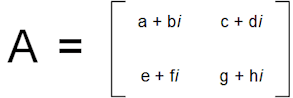and its conjugate transpose AH looks like this: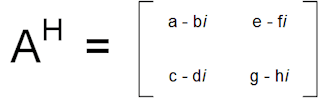If A is Skew-Hermitian, we can immediately narrow down some properties of its entries.

If we consider the top left to bottom right diagonal, then because A = – AH we have:

a + bi = – (a – bi) = – a + bi

so

a = – a

The only way that we can have a = – a for a Real Number is if a = 0, so the Real component of both the diagonal entries must be zero . So we can write: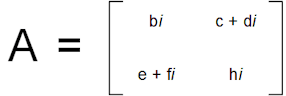Also we can note that:

c + di = – e + fi

and

– c + di = e + fi

If we add the two equations we get:

2di = 2fi

or

d = f

If we subtract them we get:

2c = – 2e

or

c = – e

So we can write our generic Skew-Hermitian matrix as: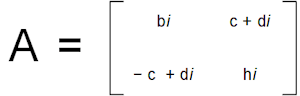Or, noting that in the above b and h stand for any Real Number and that:

– c + di = – (c + di)

we get:Where a, b ∈ ℝ and α ∈ ℂ.

From the last Chapter, We know that M3(ℝ) forms a Vector Space. Given what we know about ℝ and ℂ behaving very similarly, it is not too much of a stretch to realise that M2(ℂ) is also a Vector Space . If we pick a name for our set of 2 × 2 Skew-Hermitian matrices, say u(2), then it is easy to see that u(2) ⊂ M2(ℂ). This means that in order to show that u(2) is a Vector Space, we need only show that it is closed under vector addition and scalar multiplication.

Let’s dive in:Which is clearly also a member of u(2).

And next: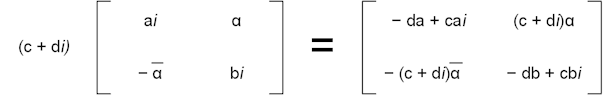But here before we try to do anything else, a problem becomes evident. We have shown that Skew-Hermitian matrices must have purely imaginary entries (ones with a real component of zero) in the top left to bottom right diagonal. Numbers of the form − da + cai where d, a, c ∈ ℝ are only purely imaginary when either d = 0 or a = 0 and not in general, so multiplying by a scalar gets us a matrix which could well not be Skew-Hermitian. What went wrong?

Well here it is worth recalling that a Vector Space is a combination of two Mathematical objects, the actual vectors, here 2 × 2 Skew-Hermitian matrices, and a Field. The choice of Field is important. Here, because the entries of our Skew-Hermitian matrices are all Complex Numbers, we have rather fallen into assuming that the scalar Field must also be the Complex Numbers. For this particular subset of M2(ℂ) using ℂ as the Field doesn’t work, however if we instead use ℝ as our Field we get:Where we set x = ca and y = cb, noting also that c = c where c ∈ ℝ. This is clearly also a Skew-Hermitian matrix and so we have shown that u(2), our set of Skew-Hermitian 2 × 2 matrices with entries in ℂ over the Field, ℝ, is a Vector Space.

The terminology used to cover this type of structure is a Real Vector Space, which implies that the scalar Field is ℝ regardless of what makes up the vectors. Equally a Complex Vector Space is one where the Field is ℂ, regardless of what makes up the vectors.

Having at first stumbled at this hurdle, but cleared it at the second attempt, we now need to consider whether or not u(2)  is a Lie Algebra as well. Having come so far, it would of course be somewhat disappointing if it was not.

So first of all we need to find our Lie Bracket, something fulfilling the role played by the cross product for ℝ3. At this point we will introduce a binary operator called the commutator, this can be defined for many different Vector Spaces. However we will be using it for Vector Spaces consisting of matrices and for this is it rather simply defined as:

For two matrices X and Y ∈ a matrix-based Vector Space, V, the commutator of X and Y is XY – YX, where XY indicates standard matrix multiplication .

Our candidate for a Lie Bracket in u(2) is thus [X, Y] = XY – YX. How does this stand up against our list of three additional properties of Lie Algebras?

1. Alternativity

Well this is pretty easy as clearly for all matrices X ∈ a Vector Space, V:

[X, X] = XX – XX = 0

So we are off to a strong start.

2. Bilinearity

Let X, Y, Z be matrices in a Vector Space, V, and f, g ∈ its Field, F, then:

[fX + gY, Z] = (fX + gY)Z – Z(fX + gY) =

fXZ + gYZ – ZfX – ZgY

because matrix multiplication is distributive over matrix addition.

But we can rearrange this as:

fXZ – ZfX + gYZ – ZgY

And also – because the order of scalar multiplication with respect to matrix multiplication is irrelevant – this can be written:

fXZ – fZX + gYZ – gYZ

Which is precisely:

f[X, Z] + g[Y, Z]

Which is what we were required to show.

Again, I’ll leave the other half of bilinearity as an exercise for the reader [hint: the same logic as above works].

3. Jacobi Identity

Here again for X, Y, Z being matrices, we have (again noting the distributivity of matrix multiplication over addition):

[X, [Y, Z]] = [X, (YZ – ZY)] = X(YZ – ZY) – (YZ – ZY)X =

XYZ – XZY – YZX + ZYX          (1)

[Z, [X, Y]] = [Z, (XY – YX)] = Z(XY – YX) – (XY – YX)Z =

ZXY – ZYX – XYZ + YXZ          (2)

[Y, [Z, X]] = [Y, (ZX – XZ)] = Y(ZX – XZ) – (ZX – XZ)Y =

YZX – YXZ – ZXY + XZY          (3)

Adding (1), (2) and (3), we get:

XYZXZYYZX + ZYX +

ZXYZYXXYZ + YXZ +

YZXYXZZXY + XZY

As in our example with the cross product, the coloured pairs team up to cancel each other out and we are left with 0.

So we have also demonstrated our third property holds.

Taken together, these results mean that u(2) is also a Lie Algebra.

 Note: The astute reader will have noticed not a single reference to the matrices discussed above being Skew-Hermitian. Indeed we have shown that the commutator forms a Lie Bracket for any set of square matrices that are also a Vector Space over a Field F. To put it the other way round, any set of square matrices that form a Vector Space is also a Lie Algebra. This is rather a useful general result, which we will rely upon in future work. In particular, this result means that, because we already know that Mn(F), the set of all n × n matrices with entries in some field F, forms a Vector Space, Mn(F) is also a Lie Algebra. When we speak of Mn(F) as a Lie Algebra, we call it gln(F); to be clear Mn(F) ≡ gln(F). This may seem a rather confusing choice of nomenclature, but it will become clearer soon. NB We have only demonstrated this result for Vector Spaces consisting of square matrices, this does not imply that every Vector Space is a Lie Algebra, in particular non-square matrices can form a Vector Space, but not a Lie Algebra. There are also Vector Spaces that do not consist of matrices.

Before we remove the first veil pertaining to the relationship between Lie Algebras and Lie Groups, let us explore a final example. This will consist of another set of matrices, one that is a subset of the Lie Algebra we have just examined. This one is equally suggestively called su(2).

A Singularly Uncommon Algebra?

We already have the Lie Algebra :By the result in the box above, any sub-Vector Space of this (a subset, which is also a Vector Space) will also be a Lie Algebra.

Let’s consider something that is obviously a subset of this by definition:This is clearly created by setting b = – a in our definition of u(2). By now the nomenclature must be arousing very significant suspicions in the minds of readers.

 Note: Without a Trace Our restriction that b = – a in the above is a specific example of something applying to the sum of the major diagonal of a matrix; the diagonal from top left to bottom right. This sum, which we met briefly in the previous Chapter, has a name in Mathematics, it is called the trace of the matrix. For a generic n × n matrix, M, with entries {ai,j}, the trace of M is written tr(M) and we have: tr(M) = a11 + a11 + … + ann The trace has a couple of properties that follow fairly obviously from its definition, including: tr(M + N) = tr(M) + tr(N) and tr(αM) = α tr(M) Where α is a scalar. For the more general Lie Algebra su(n), the definition is the Real Vector Space of n × n Skew-Hermitian matrices with a trace of zero. When n = 2, then the requirement is that the two elements making up the main diagonal are the negative of each other, hence our ai and – ai. For n = 3 then diagonals such as {0, i, – i} and {i/3, i/3, – 2i/3} would be of the right form.

To show that this is a Lie Algebra, all we need do is show that it is a sub-Vector Space, which again is established by demonstrating closure of vector addition and scalar multiplication. Given how we established both of these for u(2) above (and of course remembering that our Field is ℝ not ℂ), it is pretty evident that these criteria are satisfied and su(2) is also a Lie Algebra.

At this point an obvious question that arises is “What is the meaning of a Lie Algebra?”, is there some physical analogue to them, which helps to explain what they are? We will come back to this point in Chapter 21, but first of all we will introduce the concept of Lie Groups and how these and Lie Algebras are intimately connected; this is the subject of both the next Chapter and the following one.

 Concepts Introduced in this Chapter Lie Algebra A Vector Space which also possesses a non-associative, multiplicative binary operator, called the Lie Bracket, which satisfies the properties of alternativity, bilinearity and the Jacobi Identity (see below). Lie Bracket The above mentioned binary operator on a Vector Space, V, with Field, F, denoted by [ , ] and with the properties: Alternativity [v, v] = 0 For all v ∈ V. Bilinearity [fv + gu, w] = f[v, w] + g[u, w] and [v, fu + gw] = f[v, u] + g[v, w] For all v, u, w∈ V and f, g ∈ F. Jacobi Identity [v, [u, w]] + [w, [v, u]] + [u, [w, v]] = 0 For all v, u, w∈ V. (ℝ3, ×) The Lie Algebra consisting of Euclidean Space with the Lie Bracket provided by the cross product of coordinate vectors. Skew-Hermitian Matrices Square matrices with entries in ℂ such that their conjugate transpose is equal to the negative of the original matrix. That is n × n matrices, A, such that AH = – A. For 2 × 2 Skew-Hermitian matrices, a generic element is of the form:Where a, b ∈ ℝ and α ∈ ℂ. Real Vector Space A Vector Space whose Field is ℝ, regardless of what type of numbers form its vectors. Complex Vector Space A Vector Space whose Field is ℂ, regardless of what type of numbers form its vectors. Commutator While it has a broader definition in other areas, for n × n matrices, the formula: XY – YX, where XY denotes normal matrix multiplication. The Commutator plays the role of the Lie Bracket for square matrix Vector Spaces; all of which form Lie Algebras. u(n) The Lie Algebra consisting of n × n Skew-Hermitian matrices with the Real Numbers forming the Field of the Vector Space. su(2) The Lie Algebra consisting of the Real Vector Space of 2 × 2 Skew-Hermitian matrices of the form:Where a ∈ ℝ and α ∈ ℂ. su(n) More generally, the Lie Algebra consisting of the Real Vector Space of n × n Skew-Hermitian matrices with a trace of zero. That is if our generic matrix has entries {aij ∈ ℂ} where 1 ≤ i, j ≤ n, then we must have a11 + a22 + … + ann = 0
 Groups Discovered in this Chapter If we note that any Vector Space, V, is a Group with respect to its additive binary operator. (u(n), +) The Lie Algebra u(n) under matrix addition. (su(n), +) The Lie Algebra su(n) under matrix addition.
 < ρℝεν | ℂσητεητs | ℕεχτ >

Chapter 18 – Notes

  Both Lie Algebras and Lie Groups are named after Marius Sophus Lie, whose image appears at the top of the Chapter. Sophus Lie was a Norwegian Mathematician who pioneered this area of study. To save Sophus Lie’s blushes, it should be pointed out that celebrated Mathematician / Theoretical Physicist, Hermann Weyl, coined the phrases rather than the inventor naming his constructs after himself. Sophus Lie used the terms infinitesimal transformations and infinitesimal groups for the same things; more on the reasons why in Chapter 20.  Named after German Mathematician Carl Gustav Jakob Jacobi.  It is worth noting that the pattern of colours is the same each time, this is because all we have done is to cycle round each of v, u and w. If this is reminding you of Chapter 3, there is probably a good reason for this.  It is this property that led me to adopt the typography MH for the conjugate transpose of matrix M.  Obviously with more work.  The same argument obviously applies to g + hi.  It is actually not that tough to show that for any Field, F, the set of n × n matrices over this, or Mn(F), is also a Vector Space with respect to matrix addition.  Having gone to all that trouble to point out that the choice of Field is crucial, I am of course going to promptly stop referencing it explicitly. The reader is asked to bear in mind that for the rest of this section all scalars will be Real Numbers.  We should note here that the fact that both XY and YX are defined implies that our Vector Space needs to consist of square (i.e. n × n) matrices. Vector Spaces of matrices can include non-square matrices as the only required multiplication is scalar multiplication.  Here I use the proper Fraktur font as these are images and the rendering problem mentioned in Chapter 15 is moot.
 Text: © Peter James Thomas 2016-17. Images: © Peter James Thomas 2016-17, unless stated otherwise. Published under a Creative Commons Attribution 4.0 International License.